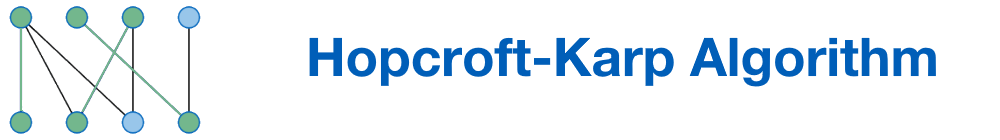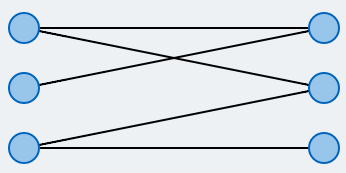How big is the maximal matching?

# Bipartite Matching

One possible application for the bipartite matching problem is allocating students to available jobs. The problem can be modeled using a bipartite graph: The students and jobs are represented by two disjunct sets of vertices. Edges represent possible assignments (based on qualifications etc). The goal is to find as many valid assignments as possible, such that each student can only take one job and each job can only be performed by a single student.

Let $$G=(V,E)$$ be a given undirected graph. A subset $$M \subseteq E$$ is called a matching, if no two edges in M share a node. A matching M is called maximal, if the cardinality of M is maximal amongst that of all matchings. In many real world problems, elements from multiple classes have to be assigned to one another. Whenever there are exactly two such classes, the problem is called bipartite matching.

## Legend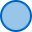Node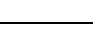Edge

## Which graph do you want to execute the algorithm on?

### Modify it to your desire:

• The graph consists of two sets of vertices and edges between these sets.
• To create a vertex in one of the sets, double click in the area of that set. You can create up to 8 nodes in each of the sets.
• To create an edge, first click on the desired origin node and then on the desired destination node.
• You can only create edges that run from one vertex-set to another.
• Right clicking will delete vertices and edges.

A occured when reading from file: the contents:

## Legend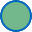matched vertexmatching edge

## Current state of the algorithm

### Initialization of the algorithm.

In the beginning, no vertex has a matching partner. Thus, the matching is empty.

In each iteration, we look for an inclusion-maximal set of shortest vertex-disjunct augmenting paths.

An augmenting path is a path that starts in an unmatched node, ends in an unmatched node and alternates between edges outside of and inside the matching.

Inclusion-maximal means that no other path can be added to the set, such that the paths found stay vertex-disjunct (i.e. don't share any vertices).

When no further augmenting paths can be found, the current matching is optimal and the algorithm terminates..

BEGIN

M := ∅

REPEAT

l := Length of the shortest

augmenting path

Ρ := {P1,...,Pk} inklusion-maximal

set of vertex-disjunct

augmenting paths of lenght l

M := M ⊕ (P1 ∪ P2 ∪ ... ∪ Pk)

UNTIL Ρ = ∅

RETURN M

END

## If you switch tabs, the execution will be terminated.

You can open another browser window to read the description in parallel.

## Legendmatched vertexmatching edge

## Test your knowledge: What would the algorithm do?

BEGIN

M := ∅

REPEAT

l := length of the shortest

augmenting path

Ρ := {P1,...,Pk} inclusion-maximal

set of vertex-disjunct

augmenting paths of length l

M := M ⊕ (P1 ∪ P2 ∪ ... ∪ Pk)

UNTIL Ρ = ∅

RETURN M

END

## In this part you can test your knowledge: What would the algorithm do?

The algorithm will be executed normally, but will stop in a few places. Then you will have to predict, what the algorithm would do next.

Hint: Recall the description of the algorithm.

## If you switch tabs, the execution will be terminated.

You can open another browser window to read the description in parallel.

## Legendmatched vertexmatching edge

## Try finding augmenting paths yourself.

The Hopcroft-Karp algorithm uses augmenting'paths in order to find a maximal matching. In this exercise you will experiment with augmenting paths.

The algorithm will stop in some places. Then you will have to fill in an augmenting path. It is not necessary that you find the shortest augmenting path. You may choose any augmenting path you like.

## Task is terminated if the tab is changed.

You can open another browser window for reading the description in parallel.